Next: MINIMUM GEOMETRIC STEINER TREE Up: Spanning Trees Previous: MINIMUM COMMUNICATION COST SPANNING   Index

### MINIMUM STEINER TREE

• INSTANCE: Complete graph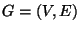, a metric given by edge weightsand a subsetof required vertices.

• SOLUTION: A Steiner tree, i.e., a subtree of G that includes all the vertices in S.

• MEASURE: The sum of the weights of the edges in the subtree.

• Good News: Approximable within.

• Comment: Variation in which the weights are only 1 or 2 is still APX-complete , but approximable within 1.28 . When all weights lie in an intervalthe problem is approximable within. On directed graphs the problem is approximable withinfor any.

The variation in which the diameter of the tree is bounded by a constant d the problem is not approximable withinfor any, unless NP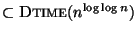, but approximable within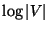forand within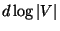for any constant. Admits a PTAS for the network Steiner tree problem if every element of S is adjacent to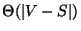elements from V-S  and .

A prize-collecting variation in which a penalty is associated with each vertex and the goal is to minimize the cost of the tree and the vertices in S not in the tree is approximable within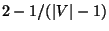. The variation, called MINIMUM K-STEINER TREE, in which an integeris given in input and at least k vertices of S must be included in the subtree is approximable within 17 . Variation in which all non-required vertices are pairwise nonadjacent is approximable within 1.28 . Variation in which there are groups of required vertices and each group must be touched by the Steiner tree is approximable within, where g is the number of groups ; when the number of groups is unbounded the problem is harder than MINIMUM DOMINATING SET to approximate, even if all edge weights are 1 .

The constrained variation in which the input is extended with a positive integer k and a subset T of E, and the problem is to find the Steiner tree of weight at most k that contains the largest number of edges from T, is not approximable within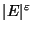for some. If the solution is allowed to be a forest with at most q trees, for a given constant q, the problem is approximable within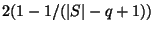. If the topology of the Steiner tree is given as input, the problem admits a PTAS . Finally, if before computing the Steiner tree the graph can be modified by reducing the weight of the edges within a given budget the problem of looking for the best reduction strategy is APX-hard .

• Garey and Johnson: ND12Next: MINIMUM GEOMETRIC STEINER TREE Up: Spanning Trees Previous: MINIMUM COMMUNICATION COST SPANNING   Index
Viggo Kann
2000-03-20